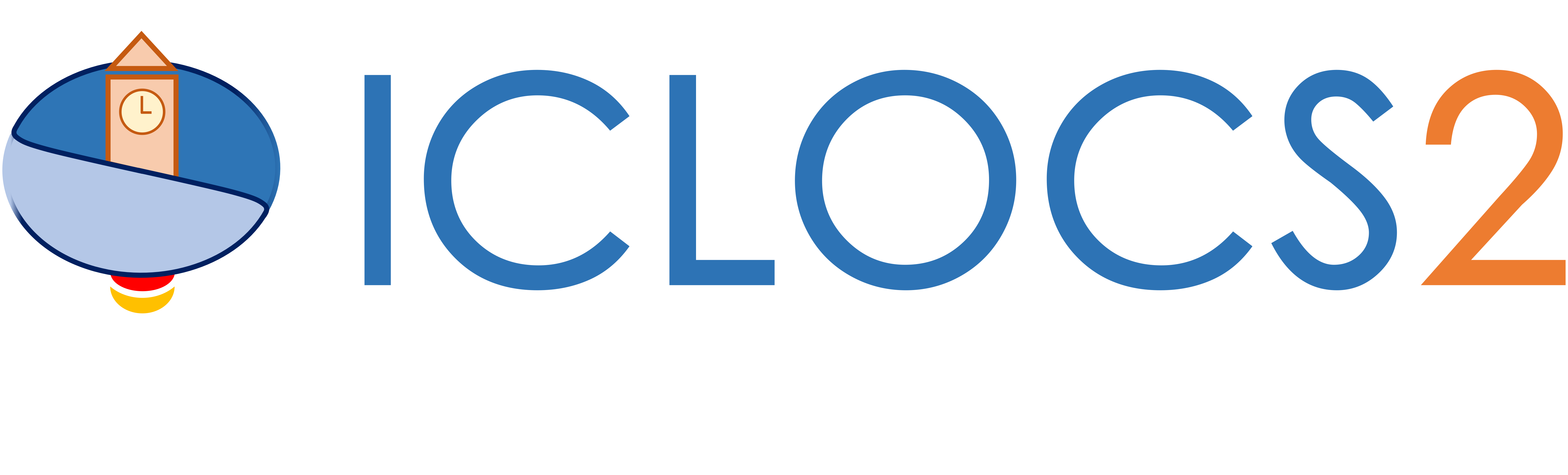Example: Goddard Rocket (Multi-Phase)

Difficulty: Hard

This problem is an extention to the single phase roddard Rocket problem. Now, we would like to solve the problem in a multi-phase formulation, and fully alleviate the influence of singular control.

Formulate the problem in ICLOCS2

Problem definition for multiphase problem

In problem defination file GoddardRocketThreePhase.m, we can define the time variables for different phases

problem.mp.time.t_min=[0 0 0 0];
problem.mp.time.t_max=[0 70 70 70];
guess.mp.time=[0 10 20 45];

the static parameters (as part of optimization variables)

problem.mp.parameters.pl=[];
problem.mp.parameters.pu=[];
guess.mp.parameters=[];

and the lower bound, uppder bound and tolerance for some linear and nonlinear linkage constraints.

problem.mp.constraints.bll.linear=[0 0 0 0 0 0 0 0];
problem.mp.constraints.blu.linear=[0 0 0 0 0 0 0 0];
problem.mp.constraints.blTol.linear=[0.01 0.01 0.01 0.01 0.01 0.01 0.01 0.01];

problem.mp.constraints.bll.nonlinear=[];
problem.mp.constraints.blu.nonlinear=[];
problem.mp.constraints.blTol.nonlinear=[];

Next, we need to specify different phases of the OCP as well as the corresponding settings for each phase

[problem.phases{1},guess.phases{1}] = GoddardRocket_Phase1(problem.mp, guess.mp);
[problem.phases{2},guess.phases{2}] = GoddardRocket_Phase2(problem.mp, guess.mp);
[problem.phases{3},guess.phases{3}] = GoddardRocket_Phase3(problem.mp, guess.mp);
phaseoptions{1}=settings_GoddardRocketThreePhase_Phase1(10);
phaseoptions{2}=settings_GoddardRocketThreePhase_Phase2(10);
phaseoptions{3}=settings_GoddardRocketThreePhase_Phase3(10);

In the linkage constraint function bclink, we can define the linkage constraint equations as

blc_linear=[xf{1}(1)-x0{2}(1);xf{1}(2)-x0{2}(2);xf{1}(3)-x0{2}(3);xf{2}(1)-x0{3}(1);xf{2}(2)-x0{3}(2);xf{2}(3)-x0{3}(3);tf(1)-t0(2);tf(2)-t0(3)];
blc_nonlinear=[];

The option file for the multiphase problem is settings_GoddardRocketThreePhase.m. The structure of the file is the same as settings_GoddardRocket.m for the single-phase problem, but only contains global settings.

Problem definition for individual phases

First, the problem definition for the individual phases can be specified following the same formulation as for single-phase problems. For this particular example, we generated the following files

• GoddardRocket_Dynamics_Phase1.m: same as GoddardRocket_Dynamics_Internal.m
• GoddardRocket_Dynamics_Phase2.m: same as GoddardRocket_Dynamics_Internal.m, with one additional path constraint from the singular arc condition
term1=T-D0.*v.^2.*exp(-h/H)-m*grav;
term2=-m*grav./(1+4*(c./v)+2*(c^2./(v.^2)));
term3=(c^2/H/grav.*(1+v./c)-1-2*c./v);
sarcconst=term1+term2.*term3;

g_eq=[sarcconst];
• GoddardRocket_Dynamics_Phase3.m: same as GoddardRocket_Dynamics_Internal.m
• GoddardRocket_Phase1.m: same as GoddardRocket.m, with minor differences. The main ones are for the time variables
% Initial time.
problem.time.t0_idx=1;
problem.time.t0_min=problem_mp.time.t_min(problem.time.t0_idx);
problem.time.t0_max=problem_mp.time.t_max(problem.time.t0_idx);
guess.t0=guess_mp.time(problem.time.t0_idx);

% Final time. Let tf_min=tf_max if tf is fixed.
problem.time.tf_idx=2;
problem.time.tf_min=problem_mp.time.t_min(problem.time.tf_idx);
problem.time.tf_max=problem_mp.time.t_max(problem.time.tf_idx);
guess.tf=guess_mp.time(problem.time.tf_idx);
for static parameters,
% Parameters bounds.
problem.parameters.pl=problem_mp.parameters.pl;
problem.parameters.pu=problem_mp.parameters.pu;
guess.parameters=guess_mp.parameters;
for input variables,
% Input bounds
problem.inputs.ul=[T_max];
problem.inputs.uu=[T_max];

% Bounds on first control action
problem.inputs.u0l=[T_max];
problem.inputs.u0u=[T_max];

% Input constraint error bounds
problem.inputs.uConstraintTol=[0.1];

% Guess the input sequences with [u0 uf]
guess.inputs(:,1)=[T_max T_max];
for the data storage static parameters, add additionally
problem.data=problem_mp.data;
and in the Mayer cost function E_unscaled
boundaryCost=0;
• GoddardRocket_Phase2.m: same as GoddardRocket.m, with minor differences. The main ones are for the time variables
% Initial time.
problem.time.t0_idx=2;
problem.time.t0_min=problem_mp.time.t_min(problem.time.t0_idx);
problem.time.t0_max=problem_mp.time.t_max(problem.time.t0_idx);
guess.t0=guess_mp.time(problem.time.t0_idx);

% Final time. Let tf_min=tf_max if tf is fixed.
problem.time.tf_idx=3;
problem.time.tf_min=problem_mp.time.t_min(problem.time.tf_idx);
problem.time.tf_max=problem_mp.time.t_max(problem.time.tf_idx);
guess.tf=guess_mp.time(problem.time.tf_idx);
for static parameters,
% Parameters bounds.
problem.parameters.pl=problem_mp.parameters.pl;
problem.parameters.pu=problem_mp.parameters.pu;
guess.parameters=guess_mp.parameters;
for boundary constraint,
problem.constraints.bl=[0 0.1];
problem.constraints.bu=[0 inf];
problem.constraints.bTol=[0.1 0.1];
for the data storage static parameters, add additionally
problem.data=problem_mp.data;
in the Mayer cost function E_unscaled
boundaryCost=0;
and in the Boundary constraint function b_unscaled
ht0 = x0(1);
vt0 = x0(2);
mt0 = x0(3);
H = vdat.H;
D0 = vdat.D0;
grav = vdat.grav;
c = vdat.c;
bc=[mt0*grav-(1+vt0/c)*D0*vt0.^2*exp(-ht0/H);tf-t0];
• GoddardRocket_Phase3.m: same as GoddardRocket.m, with minor differences. The main ones are for the time variables
% Initial time.
problem.time.t0_idx=3;
problem.time.t0_min=problem_mp.time.t_min(problem.time.t0_idx);
problem.time.t0_max=problem_mp.time.t_max(problem.time.t0_idx);
guess.t0=guess_mp.time(problem.time.t0_idx);

% Final time. Let tf_min=tf_max if tf is fixed.
problem.time.tf_idx=4;
problem.time.tf_min=problem_mp.time.t_min(problem.time.tf_idx);
problem.time.tf_max=problem_mp.time.t_max(problem.time.tf_idx);
guess.tf=guess_mp.time(problem.time.tf_idx);
for static parameters,
% Parameters bounds.
problem.parameters.pl=problem_mp.parameters.pl;
problem.parameters.pu=problem_mp.parameters.pu;
guess.parameters=guess_mp.parameters;
for input variables,
% Input bounds
problem.inputs.ul=[T_min];
problem.inputs.uu=[T_min];

% Bounds on first control action
problem.inputs.u0l=[T_min];
problem.inputs.u0u=[T_min];

% Input constraint error bounds
problem.inputs.uConstraintTol=[0.1];

% Guess the input sequences with [u0 uf]
guess.inputs(:,1)=[T_min T_min];
and for the data storage static parameters, add additionally
problem.data=problem_mp.data;
• settings_GoddardRocketThreePhase_Phase1.m: same as settings_GoddardRocket.m, but only contains phase depended settings
• settings_GoddardRocketThreePhase_Phase2.m: same as settings_GoddardRocket.m, but only contains phase depended settings
• settings_GoddardRocketThreePhase_Phase3.m: same as settings_GoddardRocket.m, but only contains phase depended settings

Main script main_GoddardRocketThreePhase.m for solving the multi-phase Goddard rocket problem

Now we can fetch the problem and options, and solve the resultant NLP.

options.mp= settings_GoddardRocketThreePhase;                  % Get options and solver settings
[problem,guess,options.phaseoptions]=GoddardRocketThreePhase;          % Fetch the problem definition
[solution,MRHistory]=solveMyProblem( problem,guess,options);

Results from ICLOCS2

Using the Hermite-Simpson discretization scheme of ICLOCS2, the following state and input trajectories are obtained under a mesh refinement scheme starting with 10 mesh points for each phase. The computation time with IPOPT (NLP convergence tol set to 1e-09) is about 2.5 seconds, using finite difference for derivative calculations on an Intel i7-6700 desktop computer.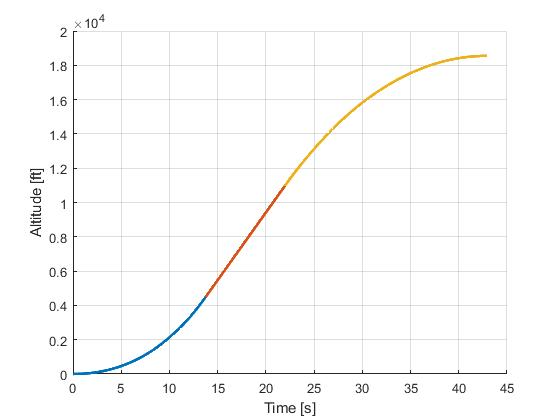Figure 1: Optimal state (altitude) trajectory for the multi-phase Goddard rocket problem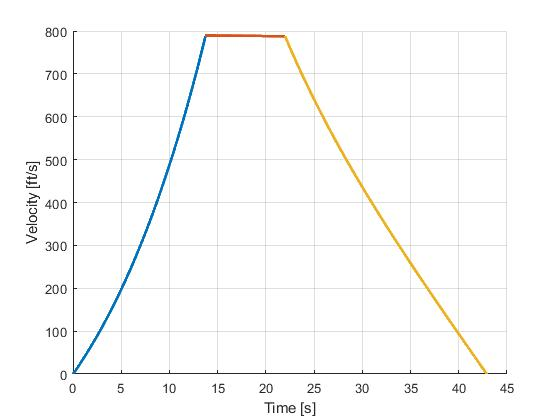Figure 2: Optimal state (velocity) trajectory for the multi-phase Goddard rocket problem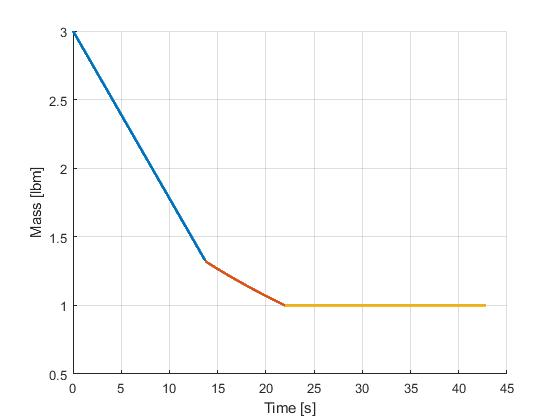Figure 3: Optimal state (mass) trajectory for the multi-phase Goddard rocket problem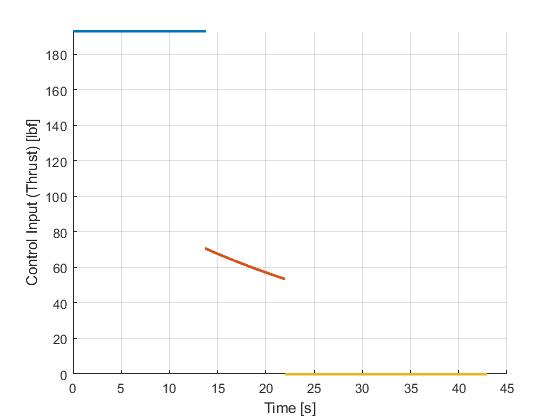Figure 4: Optimal input trajectory for the multi-phase Goddard rocket control problem

 J. Betts, "Practical Methods for Optimal Control and Estimation Using Nonlinear Programming: Second Edition," Advances in Design and Control, Society for Industrial and Applied Mathematics, 2010.